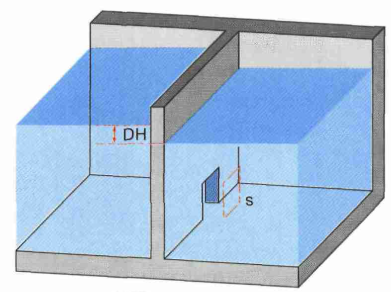# Submerged orifice formulaExcerpt from Larinier, M., Travade, F., Porcher, J.-P., Gosset, C., 1992. Passes à poissons : expertise et conception des ouvrages de franchissement. CSP. (page 94)

The equation corresponds roughly to that of the calculation module for the submerged rectangular gate with the difference that the area of the orifice is given directly rather than by the ratio of width to height:

$Q = \mu S \sqrt{2g \Delta H}$

With:

• Q the flow in m3/s;
• μ the discharge coefficient (equal to 0.7 by default);
• S the orifice surface in m2;
• ΔH the head loss H1 - H2 in m(named "Fall" in Cassiopée).